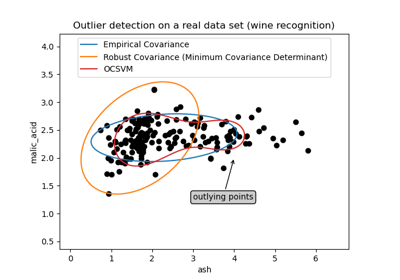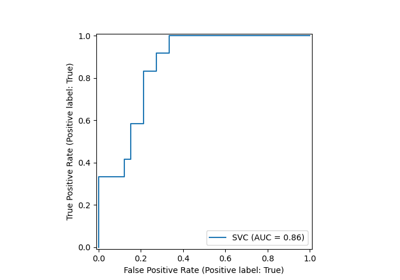# sklearn.datasets.load_wine¶

Load and return the wine dataset (classification).

New in version 0.18.

The wine dataset is a classic and very easy multi-class classification dataset.

 Classes 3 Samples per class [59,71,48] Samples total 178 Dimensionality 13 Features real, positive

The copy of UCI ML Wine Data Set dataset is downloaded and modified to fit standard format from: https://archive.ics.uci.edu/ml/machine-learning-databases/wine/wine.data

Read more in the User Guide.

Parameters:
return_X_ybool, default=False

If True, returns (data, target) instead of a Bunch object. See below for more information about the data and target object.

as_framebool, default=False

If True, the data is a pandas DataFrame including columns with appropriate dtypes (numeric). The target is a pandas DataFrame or Series depending on the number of target columns. If return_X_y is True, then (data, target) will be pandas DataFrames or Series as described below.

New in version 0.23.

Returns:
dataBunch

Dictionary-like object, with the following attributes.

data{ndarray, dataframe} of shape (178, 13)

The data matrix. If as_frame=True, data will be a pandas DataFrame.

target: {ndarray, Series} of shape (178,)

The classification target. If as_frame=True, target will be a pandas Series.

feature_names: list

The names of the dataset columns.

target_names: list

The names of target classes.

frame: DataFrame of shape (178, 14)

Only present when as_frame=True. DataFrame with data and target.

New in version 0.23.

DESCR: str

The full description of the dataset.

(data, target)tuple if return_X_y is True

A tuple of two ndarrays by default. The first contains a 2D array of shape (178, 13) with each row representing one sample and each column representing the features. The second array of shape (178,) contains the target samples.

Examples

Let’s say you are interested in the samples 10, 80, and 140, and want to know their class name.

>>> from sklearn.datasets import load_wine
>>> data.target[[10, 80, 140]]
array([0, 1, 2])
>>> list(data.target_names)
['class_0', 'class_1', 'class_2']


## Examples using sklearn.datasets.load_wine¶Outlier detection on a real data set

Outlier detection on a real data setROC Curve with Visualization API

ROC Curve with Visualization APIImportance of Feature Scaling

Importance of Feature Scaling